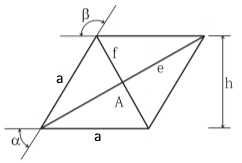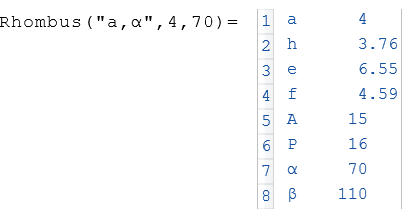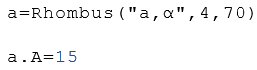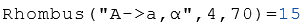# Class Rhombus

Calculation of the properties of a rhombus

## Description

The class rhombus calculates properties of a rhombus.

## Properties

The following properties can be queried as a result or passed as an argument.

#### Description

a

Lengthh

Height

A

Area

P

Perimeter

e

long diagonal

f

short diagonal

alpha

α

Angle Alpha
beta
β
Angle Beta

## Syntax

Object = Rhombus ("arg names", arguments)

Value = Rhombus ("get -> arg names", arguments)

## Example

Calculation of the properties of the side length $$a$$ and the angle $$α$$.The following example displays the area $$A$$ of the object $$a$$.Calculation of the area $$A$$ from the side length $$a$$ and the angle $$α$$.